# How to compute summary statistics

## Description

The phrase “summary statistics” usually refers to a common set of simple computations that can be done about any dataset, including mean, median, variance, and some of the others shown below.

## Solution, in Excel

View this solution alone.

Let’s assume you have some data in an Excel workbook. We show the first 10 rows the famous example dataset “iris” below, but we assume you are applying what we cover here to your own real data.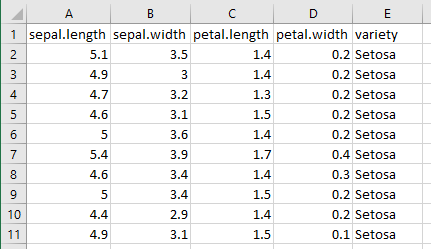To compute descriptive statistics, you will need the Data Analysis Toolpak. If you’ve never enabled it before, see these instructions from Microsoft on how to do so.

On the Data tab, click the Data Analysis button, shown below.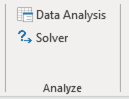From the list of tools it provides, choose Descriptive Statistics, as shown below, then click OK.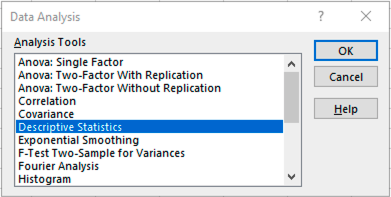Highlight as input only the numeric columns in your data, because the Data Analysis Toolpak cannot summarize other kinds of input. (Do not include column headers in your selection.) Then check the “Summary statistics” checkbox, as shown below. Then click OK.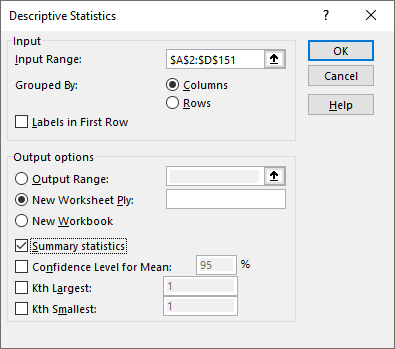Excel will create a new sheet that reports the mean, median, variance, and more, for each column, as shown below.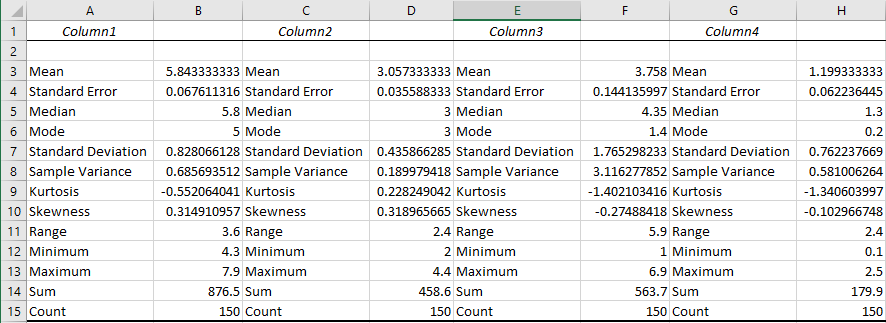To get a report of the unique values in your column and the frequency of each, you can use a pivot table. Place your cursor anywhere in your data, then on the Insert tab, choose Pivot Table, as shown below.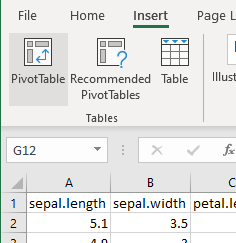Drag any column’s name from the list of pivot table fields down into both the Rows and Values areas, as shown below.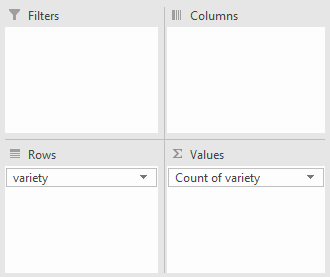If the column is numeric, then in the drop-down under Values, choose “Value field settings…” and change Sum to Count, then click OK. This is not necessary for non-numeric columns. (See how to summarize a column for an example.)

Your pivot table will now contain the desired report. In the iris dataset used in this example, there were precisely 50 observations of each variety.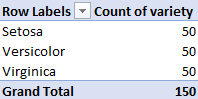See a problem? Tell us or edit the source.

## Solution, in Julia

View this solution alone.

We first load a famous dataset, Fisher’s irises, just to have some example data to use in the code that follows. (See how to quickly load some sample data.)

1
2
using RDatasets
iris = dataset( "datasets", "iris" );


How big is the dataset? The output shows number of rows then number of columns.

1
size( iris )

1
(150, 5)


What are the columns and their data types? The following command shows the first 5 rows, plus the column names and types.

1
first( iris, 5 )

5×5 DataFrame
RowSepalLengthSepalWidthPetalLengthPetalWidthSpecies
Float64Float64Float64Float64Cat…
15.13.51.40.2setosa
24.93.01.40.2setosa
34.73.21.30.2setosa
44.63.11.50.2setosa
55.03.61.40.2setosa

Are any values missing? The following command answers that question, plus provides summary statistics, and the same data type information from above.

1
describe( iris )

5×7 DataFrame
Rowvariablemeanminmedianmaxnmissingeltype
SymbolUnion…AnyUnion…AnyInt64DataType
1SepalLength5.843334.35.87.90Float64
2SepalWidth3.057332.03.04.40Float64
3PetalLength3.7581.04.356.90Float64
4PetalWidth1.199330.11.32.50Float64
5Speciessetosavirginica0CategoricalValue{String, UInt8}

The individual statistics are the column headings, and the numeric columns from the original dataset are listed under the “Symbol” heading.

We can also compute these statistics (and others) one at a time for any given set of data points. Here, we let xs be one column from the above DataFrame, but you could use any array or DataFrame instead.

1
2
3
4
5
6
7
8
9
10
11
xs = iris."SepalLength"

using Statistics

mean( xs )            # mean, or average, or center of mass
median( xs )          # 50th percentile
quantile!( xs, 0.25 ) # compute any percentile, such as the 25th
var( xs )             # variance
std( xs )             # standard deviation, the square root of the variance
sort( xs )            # data in increasing order
sum( xs )             # sum, or total


See a problem? Tell us or edit the source.

## Using pandas and NumPy, in Python

View this solution alone.

We first load a famous dataset, Fisher’s irises, just to have some example data to use in the code that follows. (See how to quickly load some sample data.)

1
2
from rdatasets import data
df = data( 'iris' )


How big is the dataset? The output shows number of rows then number of columns.

1
df.shape

1
(150, 5)


What are the columns and their data types? Are any values missing?

1
df.info()

1
2
3
4
5
6
7
8
9
10
11
12
<class 'pandas.core.frame.DataFrame'>
RangeIndex: 150 entries, 0 to 149
Data columns (total 5 columns):
#   Column        Non-Null Count  Dtype
---  ------        --------------  -----
0   Sepal.Length  150 non-null    float64
1   Sepal.Width   150 non-null    float64
2   Petal.Length  150 non-null    float64
3   Petal.Width   150 non-null    float64
4   Species       150 non-null    object
dtypes: float64(4), object(1)
memory usage: 6.0+ KB


What do the first few rows look like?

1
df.head()  # Default is 5, but you can do df.head(20) or any number.

Sepal.Length Sepal.Width Petal.Length Petal.Width Species
0 5.1 3.5 1.4 0.2 setosa
1 4.9 3.0 1.4 0.2 setosa
2 4.7 3.2 1.3 0.2 setosa
3 4.6 3.1 1.5 0.2 setosa
4 5.0 3.6 1.4 0.2 setosa

The easiest way to get summary statistics for a pandas DataFrame is with the describe function.

1
df.describe()

Sepal.Length Sepal.Width Petal.Length Petal.Width
count 150.000000 150.000000 150.000000 150.000000
mean 5.843333 3.057333 3.758000 1.199333
std 0.828066 0.435866 1.765298 0.762238
min 4.300000 2.000000 1.000000 0.100000
25% 5.100000 2.800000 1.600000 0.300000
50% 5.800000 3.000000 4.350000 1.300000
75% 6.400000 3.300000 5.100000 1.800000
max 7.900000 4.400000 6.900000 2.500000

The individual statistics are the row headings, and the numeric columns from the original dataset are listed across the top.

We can also compute these statistics (and others) one at a time for any given set of data points. Here, we let xs be one column from the above DataFrame, but you could use any NumPy array or pandas DataFrame instead.

1
2
3
4
5
6
7
8
9
10
11
xs = df['Sepal.Length']

import numpy as np

np.mean( xs )           # mean, or average, or center of mass
np.median( xs )         # 50th percentile
np.percentile( xs, 25 ) # compute any percentile, such as the 25th
np.var( xs )            # variance
np.std( xs )            # standard deviation, the square root of the variance
np.sort( xs )           # data in increasing order
np.sum( xs )            # sum, or total


See a problem? Tell us or edit the source.

## Solution, in R

View this solution alone.

We first load a famous dataset, Fisher’s irises, just to have some example data to use in the code that follows. (See how to quickly load some sample data.)

1
2
library(datasets)
data(iris)


How big is the dataset? The output shows number of rows then number of columns.

1
dim(iris)  # Short for "dimensions."

1
 150   5


What are the columns and their data types? Can I see a sample of each column?

1
str(iris)  # Short for "structure."

1
2
3
4
5
6
'data.frame':	150 obs. of  5 variables:
$Sepal.Length: num 5.1 4.9 4.7 4.6 5 5.4 4.6 5 4.4 4.9 ...$ Sepal.Width : num  3.5 3 3.2 3.1 3.6 3.9 3.4 3.4 2.9 3.1 ...
$Petal.Length: num 1.4 1.4 1.3 1.5 1.4 1.7 1.4 1.5 1.4 1.5 ...$ Petal.Width : num  0.2 0.2 0.2 0.2 0.2 0.4 0.3 0.2 0.2 0.1 ...
$Species : Factor w/ 3 levels "setosa","versicolor",..: 1 1 1 1 1 1 1 1 1 1 ...  What do the first few rows look like? 1 head(iris) # Gives 5 rows by default. You can do head(iris,10), etc.  1 2 3 4 5 6 7 Sepal.Length Sepal.Width Petal.Length Petal.Width Species 1 5.1 3.5 1.4 0.2 setosa 2 4.9 3.0 1.4 0.2 setosa 3 4.7 3.2 1.3 0.2 setosa 4 4.6 3.1 1.5 0.2 setosa 5 5.0 3.6 1.4 0.2 setosa 6 5.4 3.9 1.7 0.4 setosa  The easiest way to get summary statistics for an R data.frame is with the summary function. 1 summary(iris)  1 2 3 4 5 6 7 8 9 10 11 Sepal.Length Sepal.Width Petal.Length Petal.Width Min. :4.300 Min. :2.000 Min. :1.000 Min. :0.100 1st Qu.:5.100 1st Qu.:2.800 1st Qu.:1.600 1st Qu.:0.300 Median :5.800 Median :3.000 Median :4.350 Median :1.300 Mean :5.843 Mean :3.057 Mean :3.758 Mean :1.199 3rd Qu.:6.400 3rd Qu.:3.300 3rd Qu.:5.100 3rd Qu.:1.800 Max. :7.900 Max. :4.400 Max. :6.900 Max. :2.500 Species setosa :50 versicolor:50 virginica :50  The columns from the original dataset are the column headings in the summary output, and the statistics computed for each are listed below those headings. We can also compute these statistics (and others) one at a time for any given set of data points. Here, we let xs be one column from the above data.frame but you could use any vector or list. 1 2 3 4 5 6 7 8 9 xs <- iris$Sepal.Length

mean( xs )           # mean, or average, or center of mass
median( xs )         # 50th percentile
quantile( xs, 0.25 ) # compute any percentile, such as the 25th
var( xs )            # variance
sd( xs )             # standard deviation, the square root of the variance
sort( xs )           # data in increasing order
sum( xs )            # sum, or total